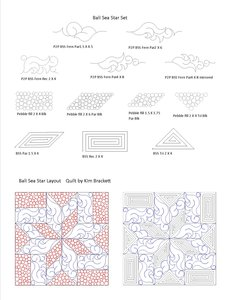# Triangles

1 Bali Sea Star SetPrice: \$40.00
Machine Type

## 60 Degree Triangle

A 60 Degree Triangle is one where all angles are the same and all sides are the same length.  It is also called an Equilateral Triangle.

## Half Square Triangle (HST)

The Half Square Triangle (HST) is one of the more basic units in quilting.  Also known as the Triangle Square, these are simply Squares made from two equal right triangles.

All of our patters the have "HST" in the name are for any block subject to density restrictions.

## Isosceles Triangles

Isosceles Triangles are ones that have have at least two equal sides

## Right Triangle

A Triangle where on corner is a Right Angle.  To use in opposite corners just Mirror the pattern